# Principal Component Analysis PCA Introduction Principal component analysis

• Slides: 20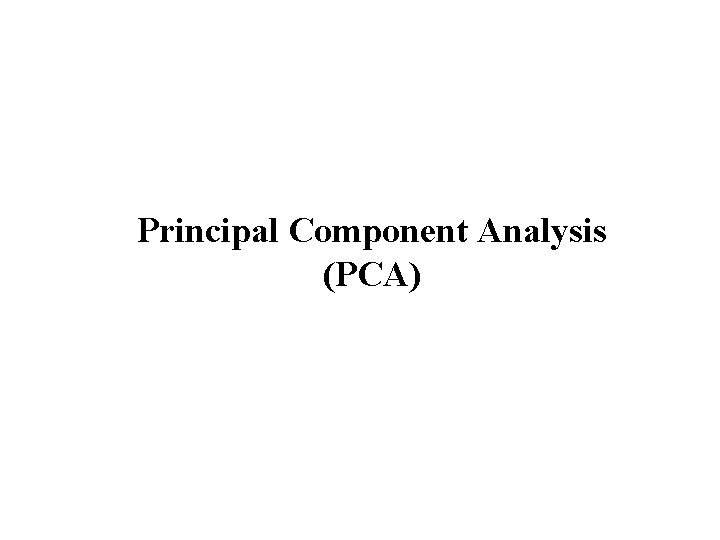Principal Component Analysis (PCA)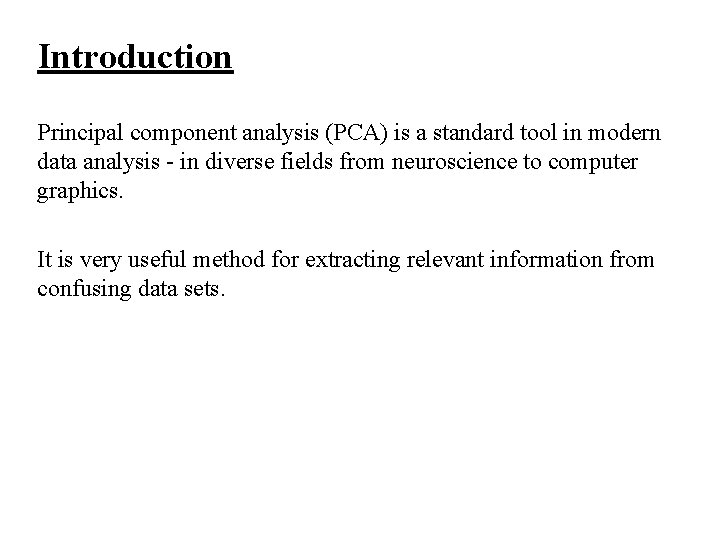Introduction Principal component analysis (PCA) is a standard tool in modern data analysis - in diverse fields from neuroscience to computer graphics. It is very useful method for extracting relevant information from confusing data sets.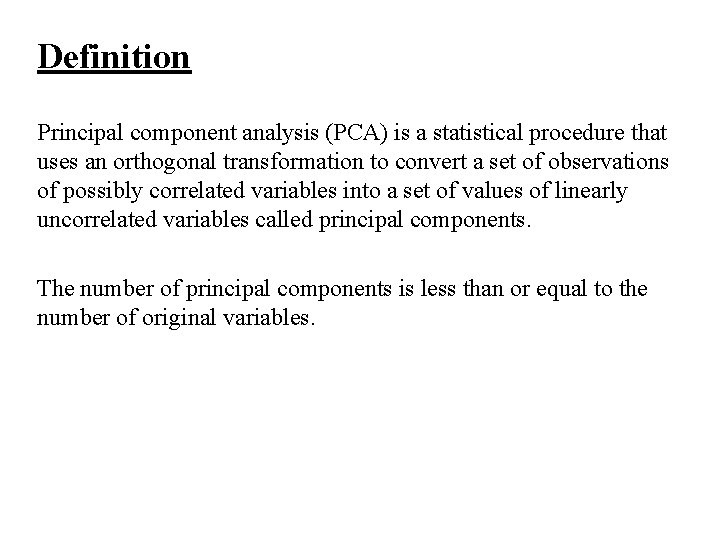Definition Principal component analysis (PCA) is a statistical procedure that uses an orthogonal transformation to convert a set of observations of possibly correlated variables into a set of values of linearly uncorrelated variables called principal components. The number of principal components is less than or equal to the number of original variables.Goals • The main goal of a PCA analysis is to identify patterns in data • PCA aims to detect the correlation between variables. • It attempts to reduce the dimensionality.Dimensionality Reduction It reduces the dimensions of a d-dimensional dataset by projecting it onto a (k)-dimensional subspace (where k<d) in order to increase the computational efficiency while retaining most of the information.Transformation This transformation is defined in such a way that the first principal component has the largest possible variance and each succeeding component in turn has the next highest possible variance.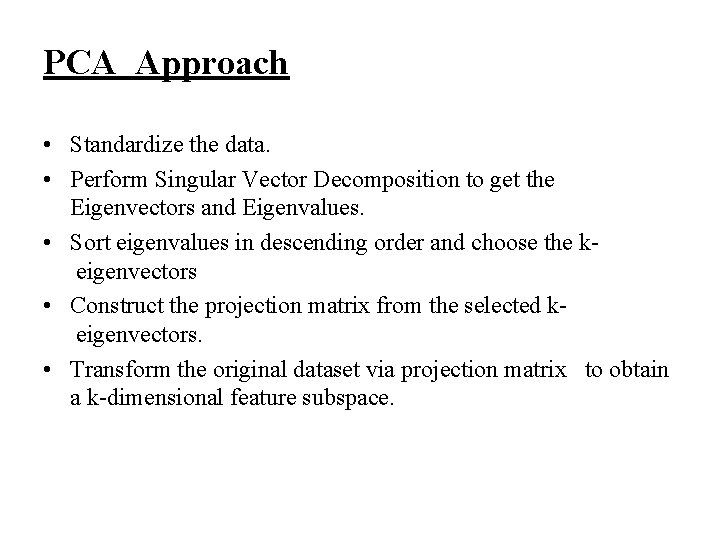PCA Approach • Standardize the data. • Perform Singular Vector Decomposition to get the Eigenvectors and Eigenvalues. • Sort eigenvalues in descending order and choose the k eigenvectors • Construct the projection matrix from the selected k eigenvectors. • Transform the original dataset via projection matrix to obtain a k-dimensional feature subspace.Limitation of PCA The results of PCA depend on the scaling of the variables. A scale-invariant form of PCA has been developed.Applications of PCA : • Interest Rate Derivatives Portfolios • Neuroscience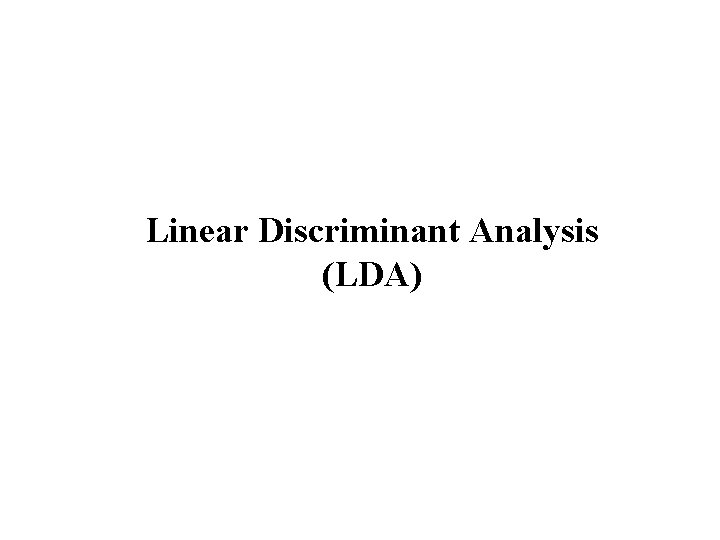Linear Discriminant Analysis (LDA)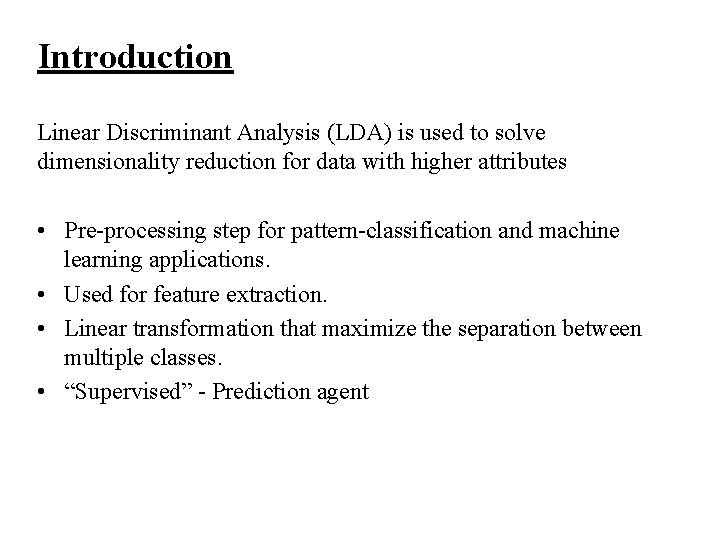Introduction Linear Discriminant Analysis (LDA) is used to solve dimensionality reduction for data with higher attributes • Pre-processing step for pattern-classification and machine learning applications. • Used for feature extraction. • Linear transformation that maximize the separation between multiple classes. • “Supervised” - Prediction agentFeature Subspace : To reduce the dimensions of a d-dimensional data set by projecting it onto a (k)-dimensional subspace (where k < d) Feature space data is well represented? • Compute eigen vectors from dataset • Collect them in scatter matrix • Generate k-dimensional data from d-dimensional dataset.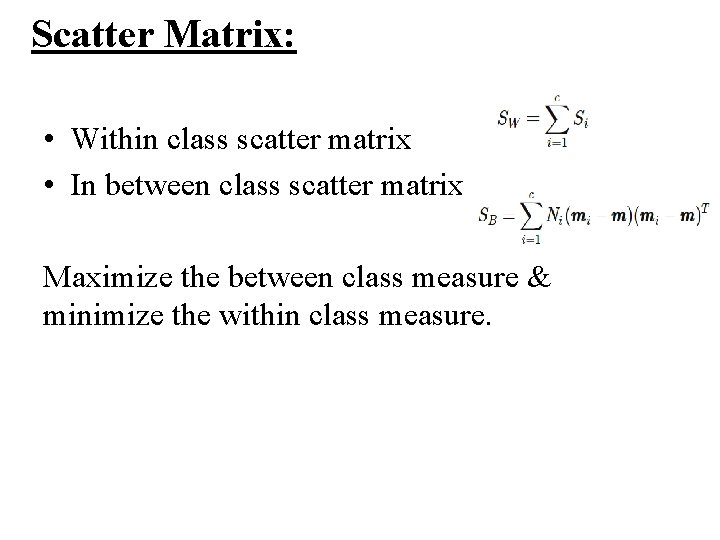Scatter Matrix: • Within class scatter matrix • In between class scatter matrix Maximize the between class measure & minimize the within class measure.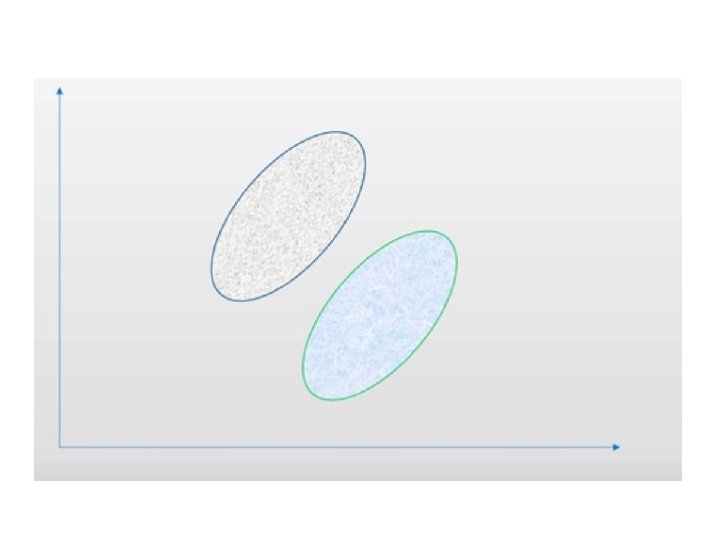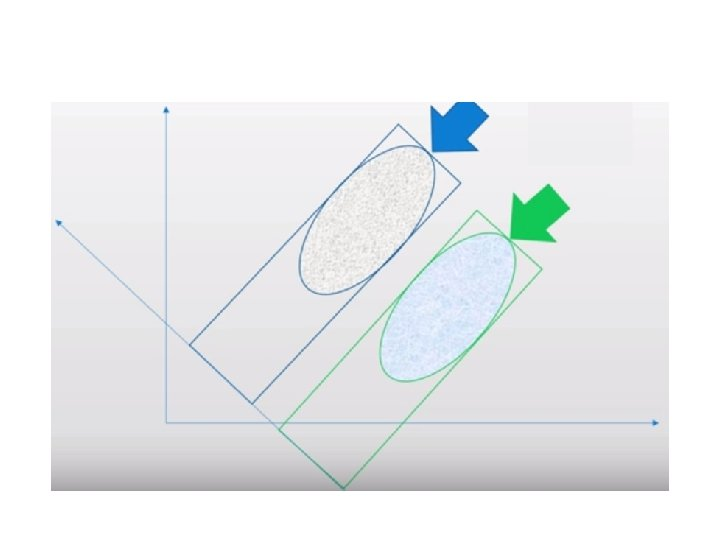LDA steps: 1. Compute the d-dimensional mean vectors. 2. Compute the scatter matrices 3. Compute the eigenvectors and corresponding eigenvalues for the scatter matrices. 4. Sort the eigenvalues and choose those with the largest eigenvalues to form a d×k dimensional matrix 5. Transform the samples onto the new subspace.Dataset Attributes : • X • O • Blank Class: • Positive(Win for X) • Negative(Win for O)Dataset topmiddle- bottom- bottomtop-lefttop-right middleleft- middle- right- Class square -square square x x o o positive x x o o o x o positive x x o o x positive o x x b o x x o o negative o x x b o x b b o negativeReferences: https: //en. wikipedia. org/wiki/Principal_component_analysis# http: //sebastianraschka. com/Articles/2015_pca_in_3_steps. ht ml#a-summary-of-the-pca-approach http: //cs. fit. edu/~dmitra/Art. Int/Project. Papers/Pca. Tutorial. pdf  Sebastian Raschka, Linear Discriminant Analysis Bit by Bit, http: //sebastianraschka. com/Articles/414_python_lda. html , 414.  Zhihua Qiao, Lan Zhou and Jianhua Z. Huang, Effective Linear Discriminant Analysis for High Dimensional, Low Sample Size Data  Tic Tac Toe Dataset - https: //archive. ics. uci. edu/ml/datasets/Tic-Tac-Toe+Endgame